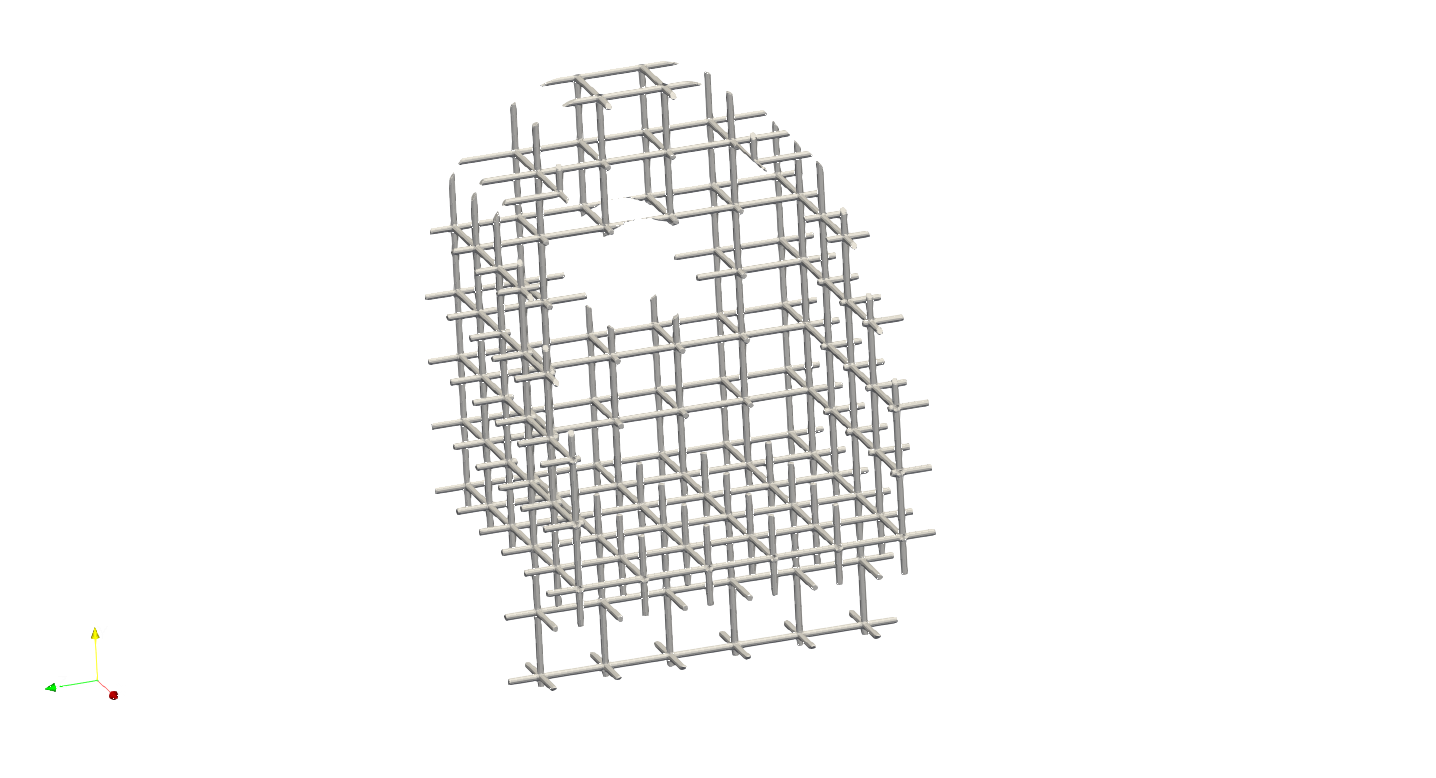# Primitive Design#

Often the design of the lightweight structure requires the lattice generation on a primitive shape, such as cylinder or sphere. Artisan can generate mesh-based discretization of the domain and morph the lattice unit onto the element. All examples in this section can be found under the folder \\Test_json\\PrimitiveDesign\\. Note that, all mesh generation of primitive shapes only supports .med file format.

## Cylindrical Domain#

The design on the cylindrical domain is common in most of industrial applications, for instance, the heat exchanger in the pressurized vessel application. Artisan integrates a simple mesh generator for the cylindrical domain. User may use this infrastructure to further produce conformal lattice. The definition of the cylindrical domain requires the radius, $$\phi$$ and height.

The following example can be find in the file GenCylindricalConformalMesh.txt.

{"Setup":{  "Type" : "Sample",
"Sample": {"Domain" : [[-10.0,10.0],[-10.0,10.0],[-10.0,10.0]], "Shape": "Box"},
"Geomfile": "",
"Rot" : [0.0,0.0,0.0],
"res":[0.1,0.1,0.1],
"onGPU": false,
"memorylimit": 1073741824000
},
"WorkFlow":{
"1": {"Gen_CylindricalMesh":{
"num_elem": [3,10,3],
"r_range": [2.0,8.0],
"phi_range": [0.0,1.0],
"ori":[0.0,0.0,-2.0],
"Height": 10.0,
"Normal": [0.0,0.0,1.0],
"Mesh_file": ".//Test_json//PrimitiveDesign//CylindricalMesh.med"
}
},
"la_name": ".//Test_json//PrimitiveDesign//GenCylindricalConformalMesh.mld",
"size": [3.0,3.0,3.0], "thk":0.25, "Rot":[0.0,0.0,0.0], "Trans":[0.0,0.0,0.0], "Inv": false, "Fill": false,
"Cube_Request": {}
}
},
"3":{
"Export": {"outfile": ".//Test_results/CylindricalMesh_ConformalLattice.stl"}
}
},
"PostProcess":{"CombineMeshes": true,
"RemovePartitionMeshFile": false,
"RemoveIsolatedParts": true,
"ExportLazPts": true}
}


The keywords Gen_CylindricalMesh contains the parameters as defined in the table below.

Parameter

Details

num_elem

it is a list parameter containing 3 elements. Each elements defines the number of lattice units in each direction.

r_range

a list parameter containing 2 elements. The first one defines the lower bound of radius, and the second one defines the upper bound of radius. Two elements forms the range of infill.

phi_range

a list parameter containing 2 elements. The first one defines the lower bound of $$\phi$$, and the second one defines the upper bound of $$\phi$$. Two elements forms the span range in $$\phi$$ direction.

ori

a list parameter defining the coordinate of origin of the cylinder shape.

Height

a float number defining the height of cylinder shape.

Normal

a list containing 3 elements, and it is the definition of the normal direction of the cylinder.

Mesh_file

the file path to store the results.

The results as blow shows a strut lattice generation on the cylinder body. User may certainly change to other types of lattice unit, e.g. TPMS or geometry.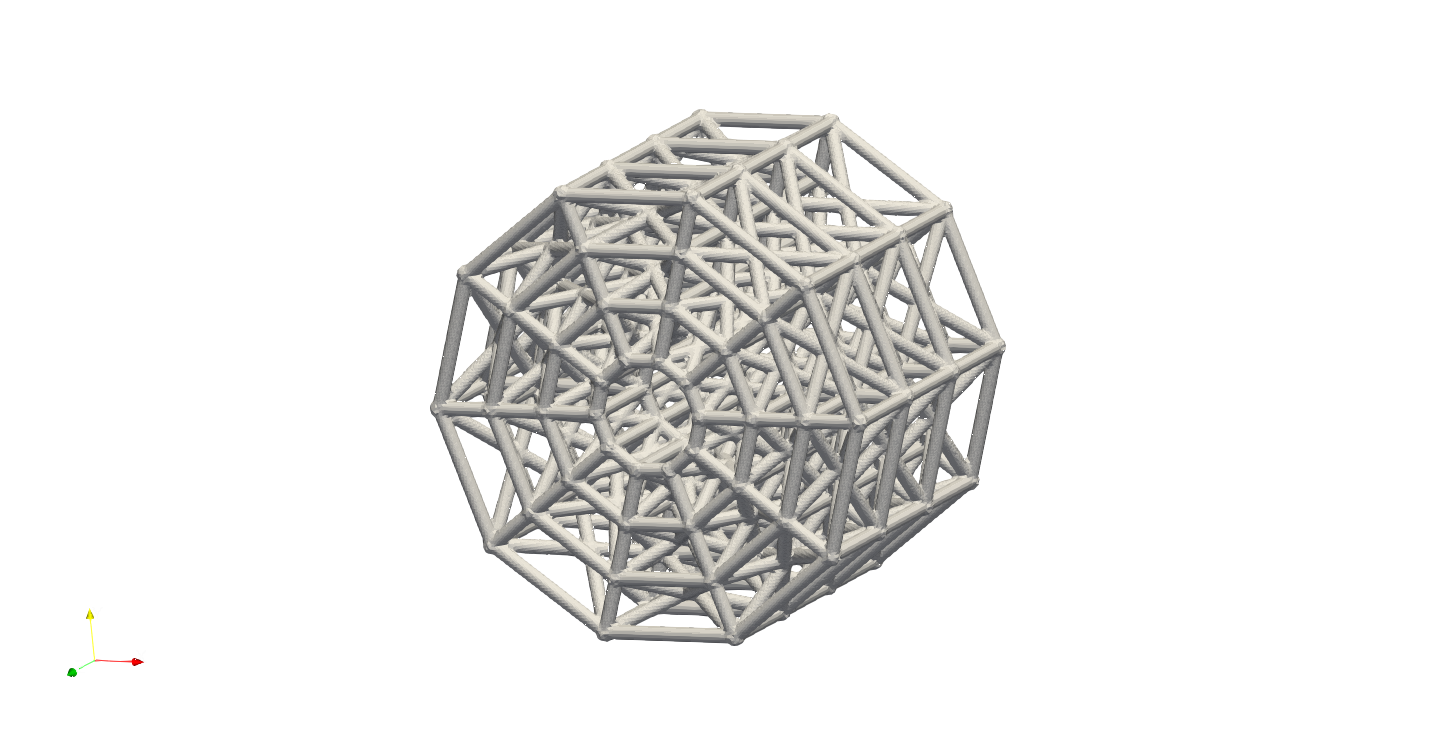The parameter phi_range provides a more interesting design features, that may help to control spanning of the cylinder. For example, the illustration below shows the case of the parameter "phi_range": [0,0.5].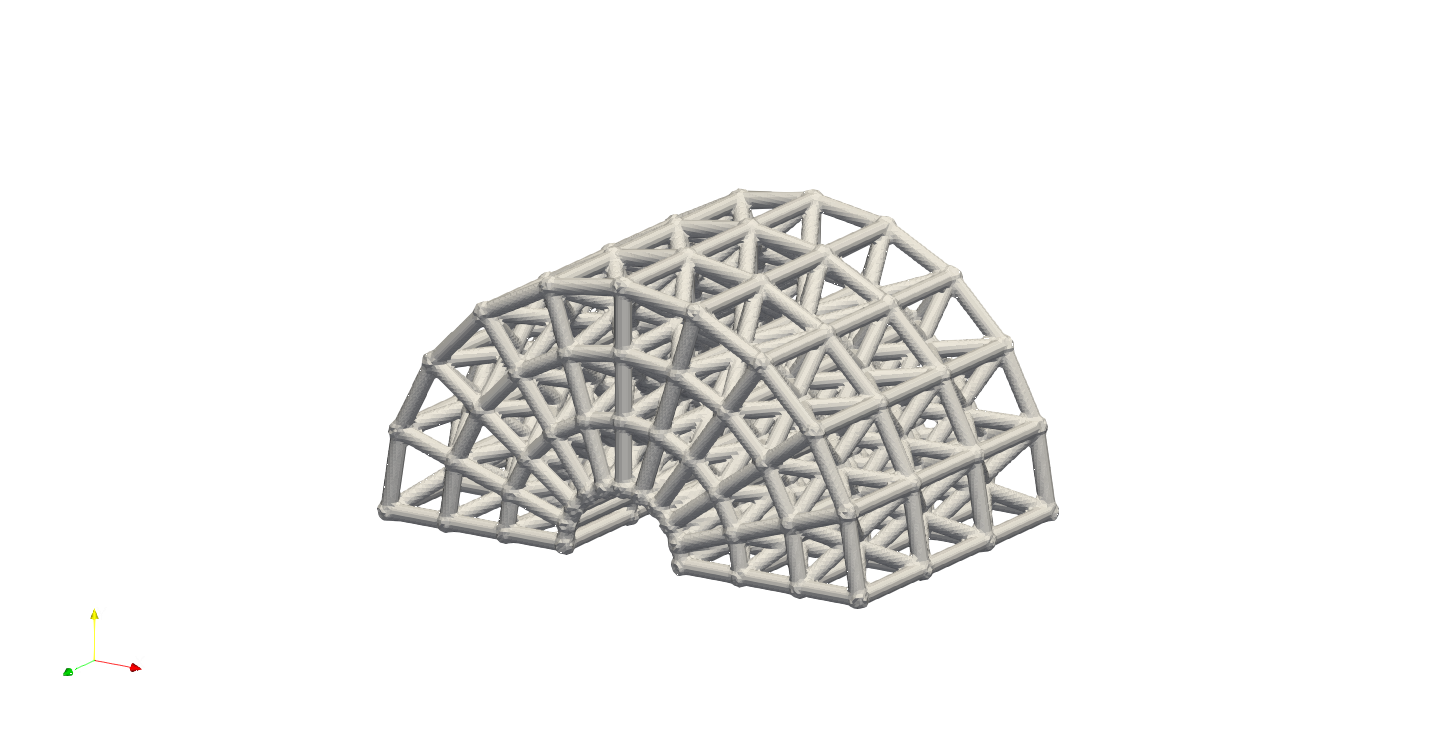## Spherical Domain#

User can generate lattices on the spherical domain through the keywords Gen_SphericalMesh. The JSON below (filename: GenSphericalConformalMesh.txt) shows a demonstration of this feature using TPMS lattice.

{"Setup":{  "Type" : "Sample",
"Sample": {"Domain" : [[-10.0,10.0],[-10.0,10.0],[-10.0,10.0]], "Shape": "Box"},
"Geomfile": "",
"Rot" : [0.0,0.0,0.0],
"res":[0.05,0.05,0.05],
"onGPU": false,
"memorylimit": 1073741824000
},
"WorkFlow":{
"1": {"Gen_SphericalMesh":{
"num_elem": [3,10,4],
"r_range": [3.0,8.0],
"phi_range": [0.0,1.0],
"theta_range":[0.3,0.7],
"ori":[0.0,0.0,0.0],
"Normal": [1.0,1.0,0.0],
"Mesh_file": ".//Test_json//PrimitiveDesign//SphericalMesh.med"
}
},
"la_name": ".//Test_json//PrimitiveDesign//GenSphericalConformalMesh.mld",
"size": [3.0,3.0,3.0], "thk":0.2, "Rot":[0.0,0.0,0.0], "Trans":[0.0,0.0,0.0], "Inv": false, "Fill": false,
"Cube_Request": {}
}
},
"3":{
"Export": {"outfile": ".//Test_results/SphericalMesh_ConformalLattice.stl"}
}
},
"PostProcess":{"CombineMeshes": true,
"RemovePartitionMeshFile": false,
"RemoveIsolatedParts": true,
"ExportLazPts": true}
}


The parameters in Gen_SphericalMesh are explained in the table below.

Parameter

Details

num_elem

it is a list parameter containing 3 elements. Each elements defines the number of lattice units in each direction.

r_range

a list parameter containing 2 elements. The first one defines the lower bound of radius, and the second one defines the upper bound of radius. Two elements forms the range of infill.

phi_range

a list parameter containing 2 elements. The first one defines the lower bound of $$\phi$$, and the second one defines the upper bound of $$\phi$$. Two elements forms the span range in $$\phi$$ direction.

theta_range

a list parameter containing 2 elements. The first one defines the lower bound of $$\theta$$, and the second one defines the upper bound of $$\phi$$. Two elements forms the span range in $$\theta$$ direction.

ori

a list parameter defining the coordinate of origin of the cylinder shape.

Height

a float number defining the height of cylinder shape.

Normal

a list containing 3 elements, and it is the definition of the normal direction of the cylinder.

Mesh_file

the file path to store the results.

Below presented the resultant lattice on spherical domain.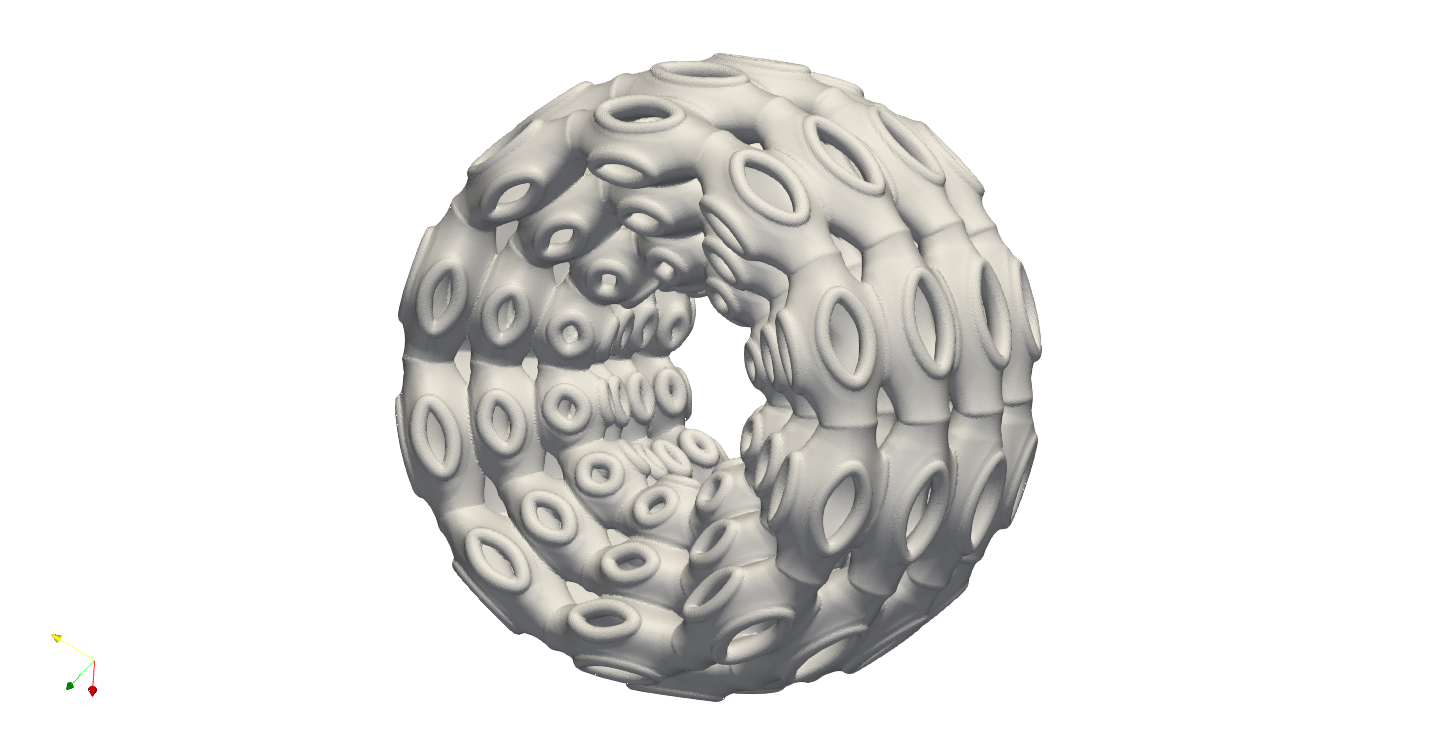Similar to the Gen_CylindricalMesh, the keywords Gen_SphericalMesh can also change the spanning of domain. In the case above, we have a complete $$\phi$$ direction spanning, and the spanning of $$\theta$$ is between 0.3 to 0.7 - $$0.3\times\pi$$ to $$0.7\times\pi$$. User may alter both phi_range and theta_range to generate various combined spanning of the domain. The illustration below shows the case with parameter value "phi_range":[0.2, 0.8].## Box Domain#

User may define a hex mesh in the box-shape domain, and then conformal the lattice structure. This will produce the exact same results as the conventional way of lattice filling in the domain. The conformal lattice uses a different algorithm to compute the infill to the conventional periodic lattice infill. The base code of conformal lattice use openMP to accelerate the calculations, this can be dramatically faster when number of infill is huge. This functionality aims to provide an easy access to the faster algorithm in order to improve the efficiency. Below shows an example of filling lattice using the generated box mesh. The example file can be found at \\PrimitiveDesign\\GenBoxConformalMesh.txt.

{"Setup":{  "Type" : "Sample",
"Sample": {"Domain" : [[0.0,20.0],[0.0,20.0],[0.0,20.0]], "Shape": "Box"},
"Geomfile": "",
"Rot" : [0.0,0.0,0.0],
"res":[0.05,0.05,0.05],
"onGPU": false,
"memorylimit": 1073741824000
},
"WorkFlow":{
"1": {"Gen_BoxMesh":{
"num_elem": [8,8,8],
"x_range": [0.0, 15.0],
"y_range": [0.0, 15.0],
"z_range": [0.0, 15.0],
"ori":[0.0,0.0,0.0],
"Normal": [0.0,0.0,1.0],
"Mesh_file": ".//Test_json//PrimitiveDesign//BoxMesh.med"
}
},
"la_name": ".//Test_json//PrimitiveDesign//GenBoxConformalMesh.mld",
"size": [2.5,2.5,2.5], "thk":0.1, "Rot":[0.0,0.0,0.0], "Trans":[0.0,0.0,0.0], "Inv": false, "Fill": false,
"Cube_Request": {}
}
},
"3":{
"Export": {"outfile": ".//Test_results/BoxMesh_ConformalLattice.stl"}
}
},
"PostProcess":{"CombineMeshes": true,
"RemovePartitionMeshFile": false,
"RemoveIsolatedParts": false,
"ExportLazPts": false}
}


The result, as expected, is lattice infill in a box.Here is the explanation of parameters.

Parameter

Details

num_elem

it is a list parameter containing 3 elements. Each elements defines the number of lattice units in each direction.

x_range

a list parameter containing 2 elements. The lower and upper bounds of the in x direction - the direction before rotation.

y_range

a list parameter containing 2 elements. The lower and upper bounds of the in y direction - the direction before rotation.

z_range

a list parameter containing 2 elements. The lower and upper bounds of the in z direction - the direction before rotation.

ori

a list parameter defining the coordinate of origin of the cylinder shape.

Normal

a list containing 3 elements, and it is the definition of the normal direction of the box. If the direction is not aligned with [0.0, 0.0, 1.0], the box will be rotated accordantly.

Mesh_file

the file path to store the results.

## Primitive Shape and Geometry handling#

A few simple geometry handling functions have been integrated into Artisan. It could be useful if adding some geometric entity after lattice infill. It has to mention that, the whole geometry handling is based on implicit modelling concept, explicit modelling capability is not available. In general, user may Add, Subtract and Intersect on the shape Sphere, Box, Cylinder and other user defined geometries.

In the example file \\Test_json\\PrimitiveDesign\\GenBox.txt, a simple geometry was constructed using Sphere, Box, Cylinder.

 {"Setup":{    "Type" : "Sample",
"Sample": {"Domain" : [[-5.0,5.0],[-5.0,5.0],[-5.0,5.0]], "Shape": "Box"},
"Geomfile": "",
"Rot" : [0.0,0.0,0.0],
"res":[0.05,0.05,0.05],
"onGPU": false,
"memorylimit": 1073741824000
},
"WorkFlow":{
"Name": "Box",
"k_factor": 0.0,
"push2GeomField": false,
"Paras": {
"ori": [0.0, 0.0, 0.0],
"normal": [0, 0, 1],
"length": [2, 2, 2]
}
}
},
"Name": "Cylinder",
"k_factor": 0.7,
"push2GeomField": false,
"Paras": {
"pa": [0.0, 0.0, 0.0],
"pb": [0.0, 0.0, 5.0],
"r": 1.0
}
}
},
"3": {"Subtract_Geometry":{
"Name": "Sphere",
"k_factor": 0.5,
"push2GeomField": false,
"Paras": {
"ori": [-2.0, 0.0, 0.0],
"r": 1.5
}
}
},
"4":{
"Export": {"outfile": ".//Test_results/GenBox.stl"}
}
},
"PostProcess":{"CombineMeshes": true,
"RemovePartitionMeshFile": false,
"RemoveIsolatedParts": false,
"ExportLazPts": false}
}


Above JSON will produce a result of two combined shape with a subtracted spherical indent.There are other three examples in the folder \\Test_json\\, called GenBox_Add.txt, GenBox_Subtract.txt and GenBox_Intersection.txt. Three examples demonstrates the different operations between two boxes. Below shows the overlapping of three results. The red part is intersection part, the blue and grey makes the adding union results, and the grey is the cut result. All operation used the smooth transitions.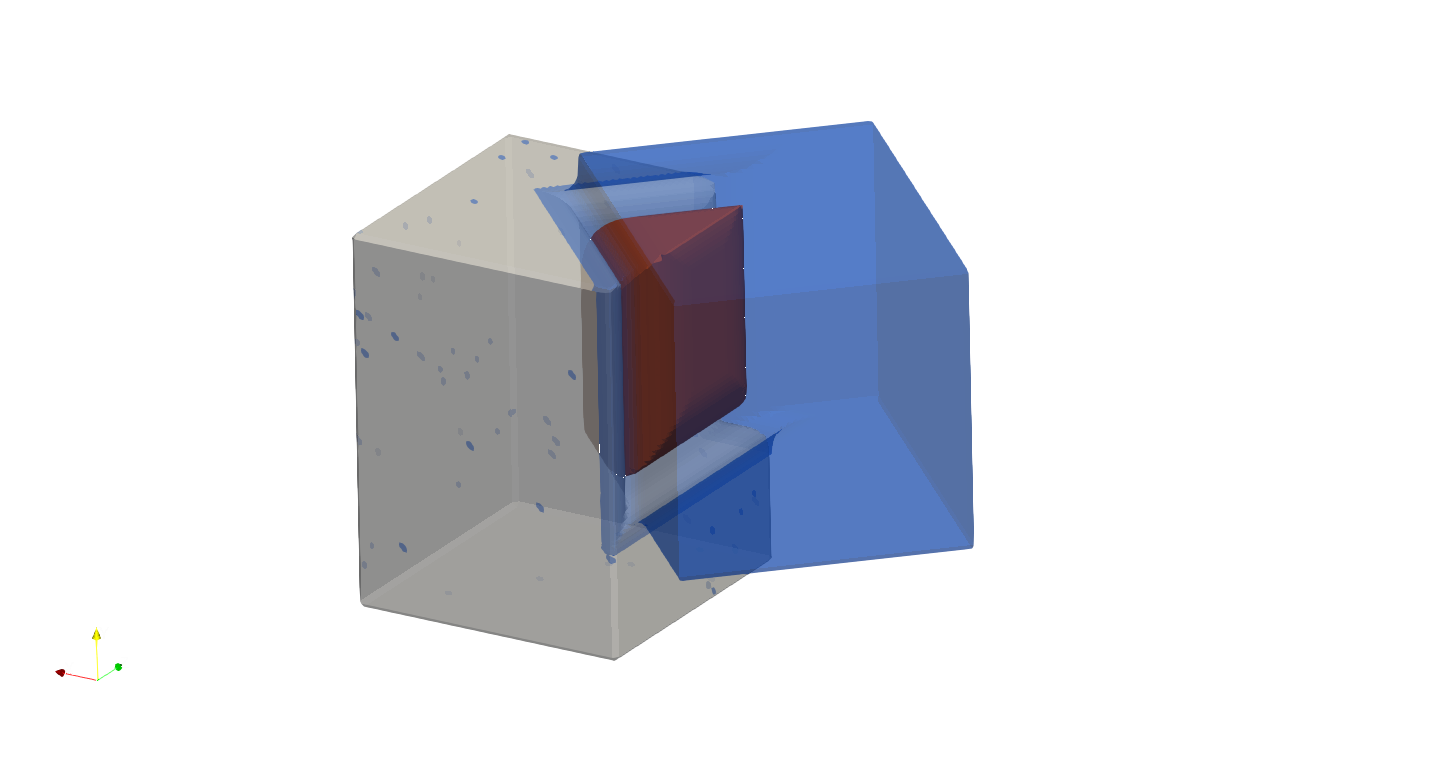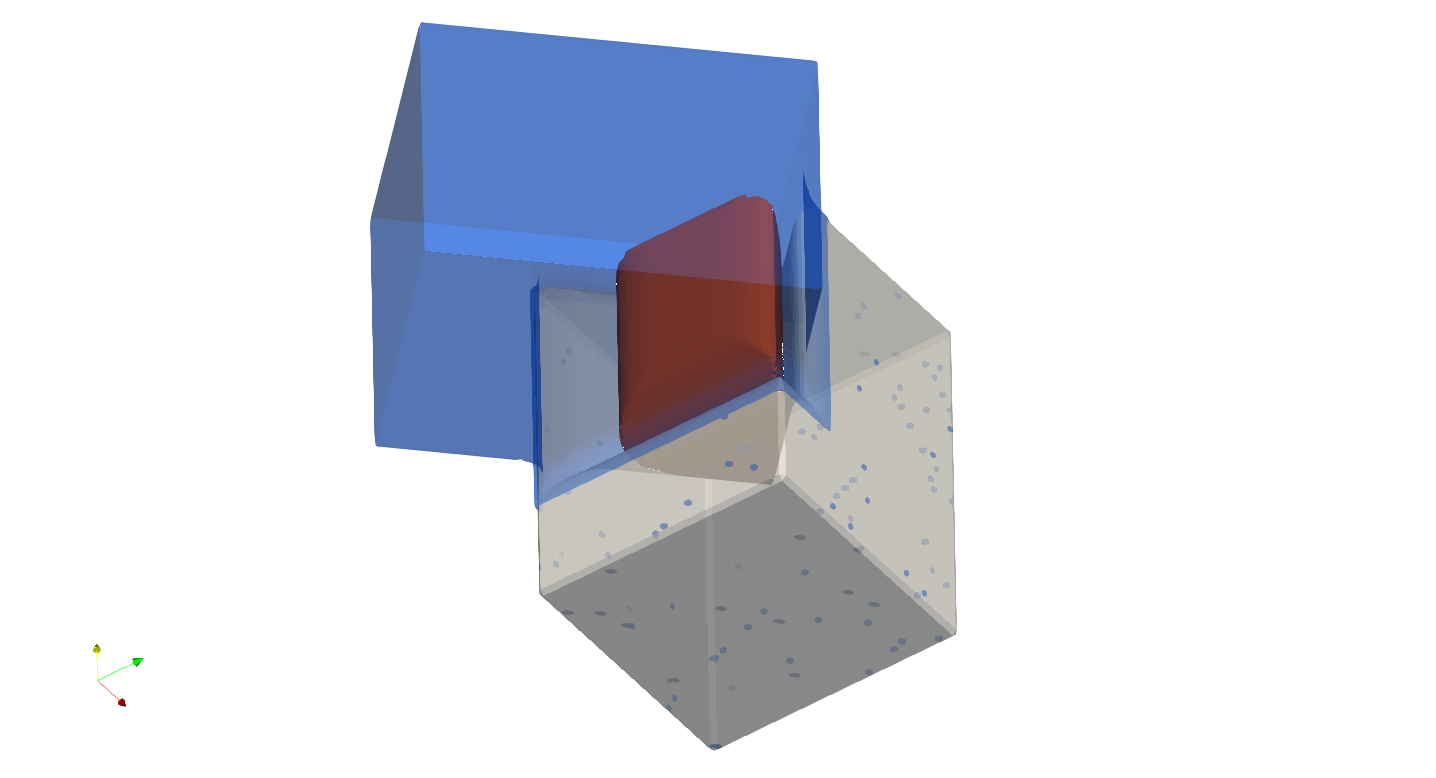The keywords Add_Geometry and Subtract_Geometry manipulate the geometry handling, such as adding and subtracting. Along with the keywords Intersect_Geometry, three keywords shared same parameters.

Parameter

Details

Name

"Sphere", "Box", "Cylinder" or the file path to the geometry file.

k_factor

A float number above 0.0. It represents the smoothness transition around the conjunction areas. If 0.0, no smoothness. It should be a number less than 1.0 in most of cases, but may beyond 1.0.

"push2GeomField"

If true, the geometry handling will be applied to geometric field. If false, the operation is applied to lattice field.

"Paras"

The parameters used for the primitive shapes and geometries.

The Box shape has following parameters:

Parameter

Details

ori

The center point coordinate of the box.

normal

A directional vector which forms the rotation from [0.0, 0.0, 1.0] to this given vector. If it is aligned with [0.0, 0.0, 1.0], no rotation.

The Sphere shape has following parameters:

Parameter

Details

ori

The center point coordinate of the sphere.

r

The Cylinder shape has following parameters:

Parameter

Details

pa

The center point coordinate of the box.

pb

A directional vector which forms the rotation from [0.0, 0.0, 1.0] to this given vector. If it is aligned with [0.0, 0.0, 1.0], no rotation.

r

The radius of the cylindrical body.

It is possible to load external geometry, as demonstrated below. The JSON shows the parameter Name in the keyword Add_Geometry became a file path string. It is interpreted as an external geometry, not an integrated primitive shape.

{"Setup":{    "Type" : "Sample",
"Sample": {"Domain" : [[-10.0,10.0],[-10.0,10.0],[-10.0,10.0]], "Shape": "Box"},
"Geomfile": "",
"Rot" : [0.0,0.0,0.0],
"res":[0.05,0.05,0.05],
"onGPU": false,
"memorylimit": 1073741824000
},
"WorkFlow":{

"Name": ".//sample-obj//Klemmwinkel Form A.stl",
"k_factor": 0.0,
"push2GeomField": true,
"Paras": {
"Scale": [0.5, 0.5, 0.5],
"Trans": [0.0, 0.0, 5.0],
"Rot": [0, 0, 0]
}
}
},
"la_name": "Cubic", "size": [2.0,2.0,2.0], "thk":0.1,
"Rot":[0.0, 0.0, 0.0], "Trans":[0.0, 0.0, 0.0],
"Inv": false, "Fill": true,
"Cube_Request": {}
}
},
"3":{
"Export": {"outfile": ".//Test_results/GenGeom.stl"}
}
},
"PostProcess":{"CombineMeshes": true,
"RemovePartitionMeshFile": false,
"RemoveIsolatedParts": true,
"ExportLazPts": true}
}


In this case, parameter Paras has the following operation parameters, i.e. Scale, Trans and Rot. The operation sequences is scale, translation, then rotation.

Parameter

Details

Scale

a list containing 3 elements, representing scales in three dimensions.

Trans

a list containing 3 elements, translational vector before rotation.

Rot

a list of three radiuses defining the rotation using x, y and z axis.

The JSON reads an external geometry, conducted scale and translation, and push to geometric field, then conduct the periodic lattice infill in the geometry.